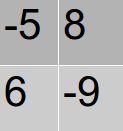Monk and Operations
Tag(s):

## Data Structures, Easy, approved

Problem
Editorial
Analytics

Monk is fond of matrices. Today, he created a matrix A of size $N \times M$. He defines four types of operations on the matrix as follows:

1. Add $v1$ to all elements of a row.

2. Update the value of all elements of a row to $v2$, i.e., all elements of that row become equal to $v2$.

3. Add $v3$ to all elements of a column.

4. Update the value of all elements of a column to $v4$, i.e., all elements of that column become equal to $v4$.

He defines a function $F(x)$ as

$F(x)$ = $\sum_{i=1}^{N} \sum_{j=1}^{M} abs(A[i][j])$

where $A[i][j]$ refers to the $j^{th}$ cell in the $i^{th}$ row of matrix A, and $abs(x)$ refers to absolute value of any integer x.

Now, he has defined some restrictions:

1. On any cell of the matrix, at most one operation can be performed. This operation can be of any type.

2. Any type of operation can be used any number of times.

You need to maximize the value of $F(x)$ following these restrictions.

Input Format:

The first line consists of two integers N and M, denoting the number of rows and number of columns in matrix A respectively.

Each of the following N lines consist of M space-separated integers, where the $j^{th}$ integer in the $i^{th}$ line denotes $A[i][j]$.

The last line of input consists of four space-separated integers denoting the values of $v1$,$v2$,$v3$ and $v4$ respectively.

Output Format:

The only line of output should consist of the maximum value of $F(x)$.

Input Constraints:

$1 \le N \le 1000$

$1 \le M \le 1000$

$-10^9 \le A[i][j] \le 10^9$

$-10^9 \le v1,v2,v3,v4 \le 10^9$

SAMPLE INPUT
2 2
-5 8
6 -9
-2 5 -1 6

SAMPLE OUTPUT
29

ExplanationWe use third type of operation on column 2 (or we can leave column 2 as it is) and fourth type of operation on column 1.
So, $F(x)$ = $abs(6)+abs(8-1)+abs(6)+abs(-9-1)$ = $6+7+6+10$ = $29$

Time Limit: 1.0 sec(s) for each input file.
Memory Limit: 256 MB
Source Limit: 1024 KB
Marking Scheme: Marks are awarded when all the testcases pass.
Allowed Languages: Bash, C, C++, C++14, Clojure, C#, D, Erlang, F#, Go, Groovy, Haskell, Java, Java 8, JavaScript(Rhino), JavaScript(Node.js), Julia, Kotlin, Lisp, Lisp (SBCL), Lua, Objective-C, OCaml, Octave, Pascal, Perl, PHP, Python, Python 3, R(RScript), Racket, Ruby, Rust, Scala, Swift, Swift-4.1, TypeScript, Visual Basic

## CODE EDITOR

Initializing Code Editor...

## This Problem was Asked inChallenge Name

CodeMonk (Checkpoint - I)

OTHER PROBLEMS OF THIS CHALLENGE
• Data Structures > Arrays
• Algorithms > Searching
• Data Structures > Stacks
• Math > Number Theory
• Data Structures > Stacks